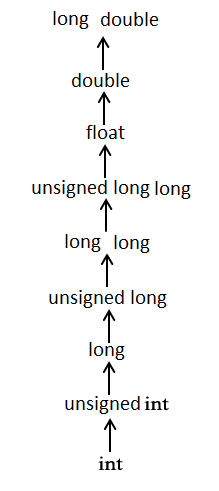# C 强制类型转换

2018-12-09 15:13 更新

## C 强制类型转换

```(type_name) expression
```

``````#include <stdio.h>

int main()
{
int sum = 17, count = 5;
double mean;

mean = (double) sum / count;
printf("Value of mean : %f\n", mean );

}
``````

```Value of mean : 3.400000
```

## 整数提升

``````#include <stdio.h>

int main()
{
int  i = 17;
char c = 'c'; /* ascii 值是 99 */
int sum;

sum = i + c;
printf("Value of sum : %d\n", sum );

}
``````

```Value of sum : 116
```

## 常用的算术转换``````#include <stdio.h>

int main()
{
int  i = 17;
char c = 'c'; /* ascii 值是 99 */
float sum;

sum = i + c;
printf("Value of sum : %f\n", sum );

}
``````

```Value of sum : 116.000000
```

## 您可能还喜欢：

App下载